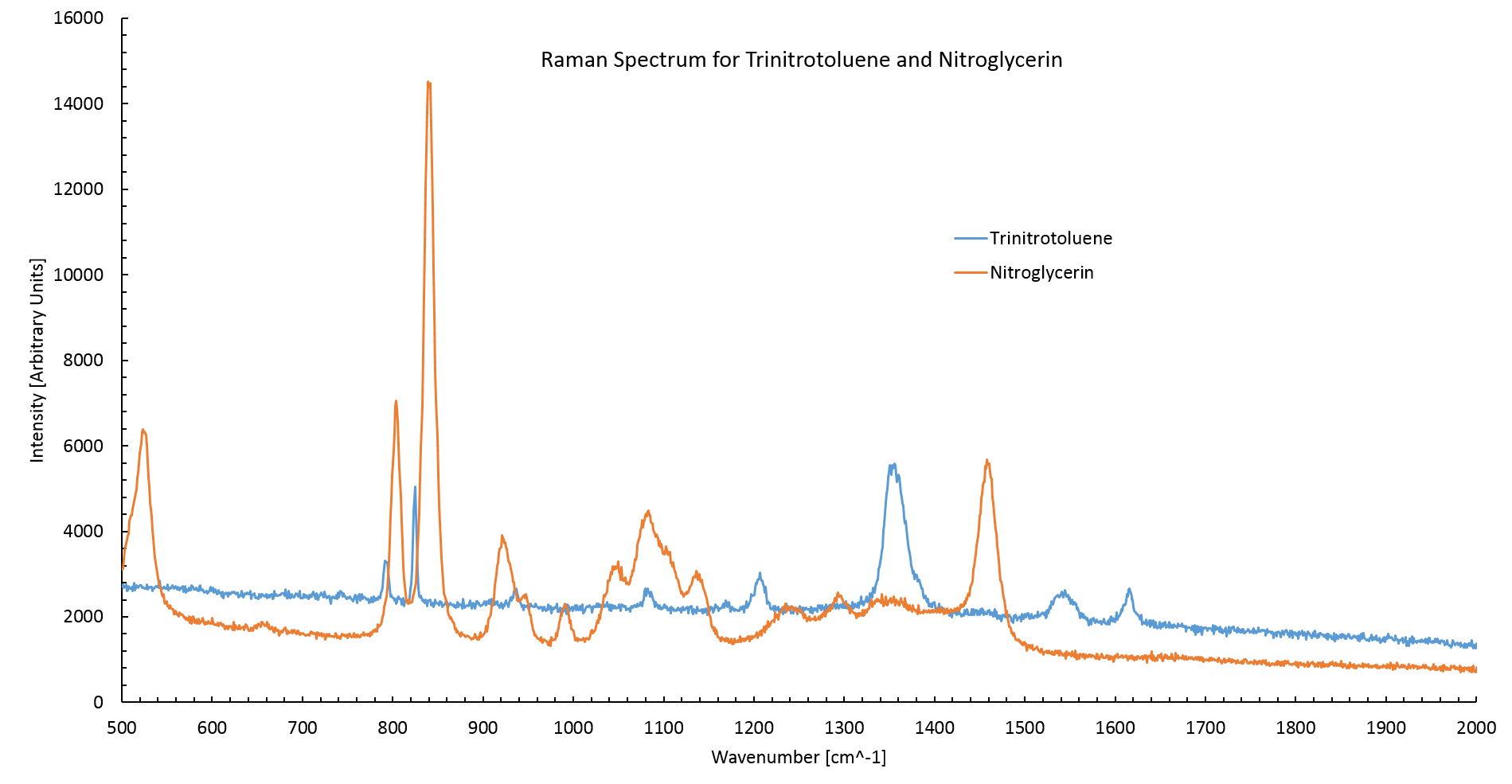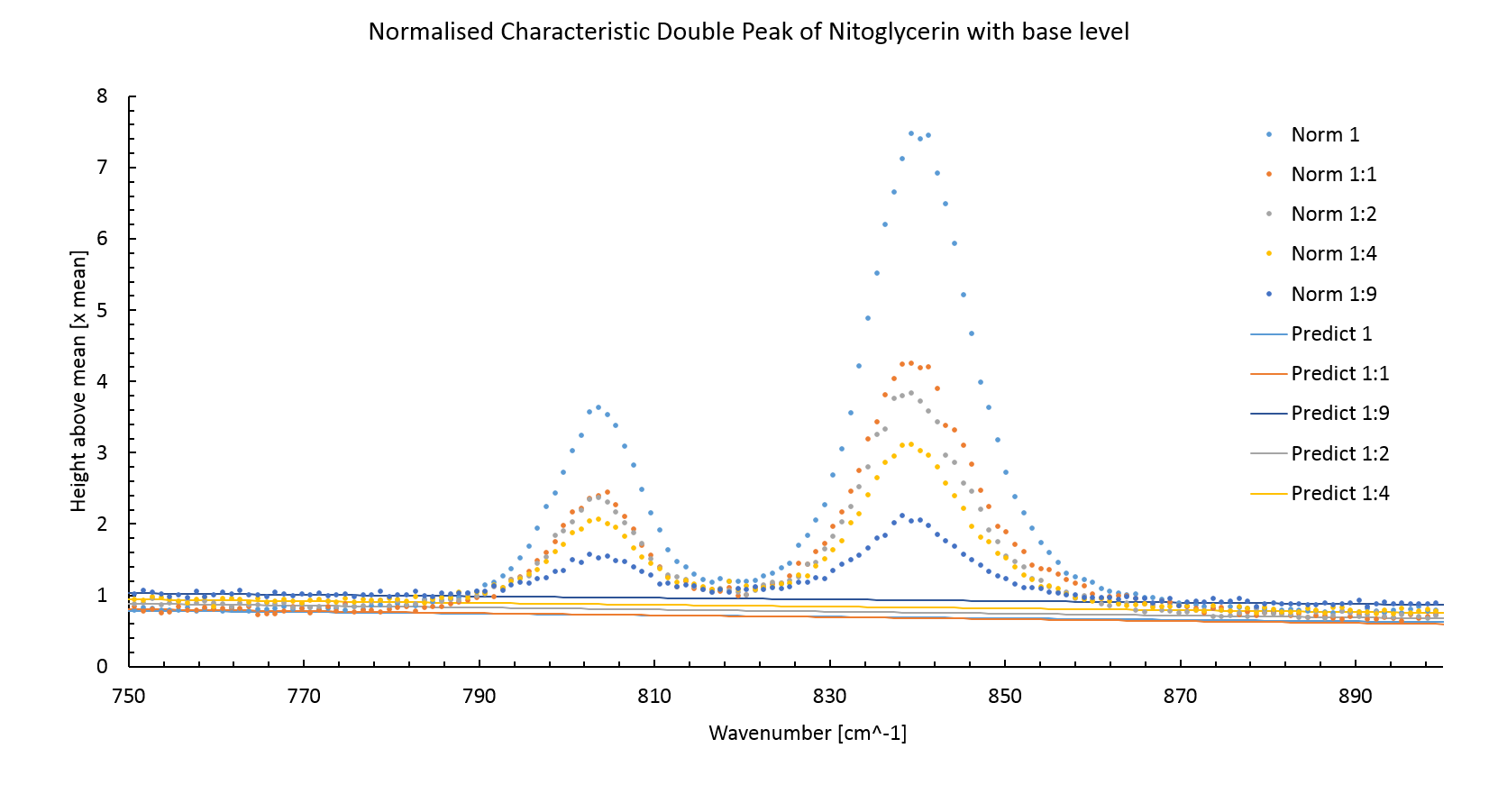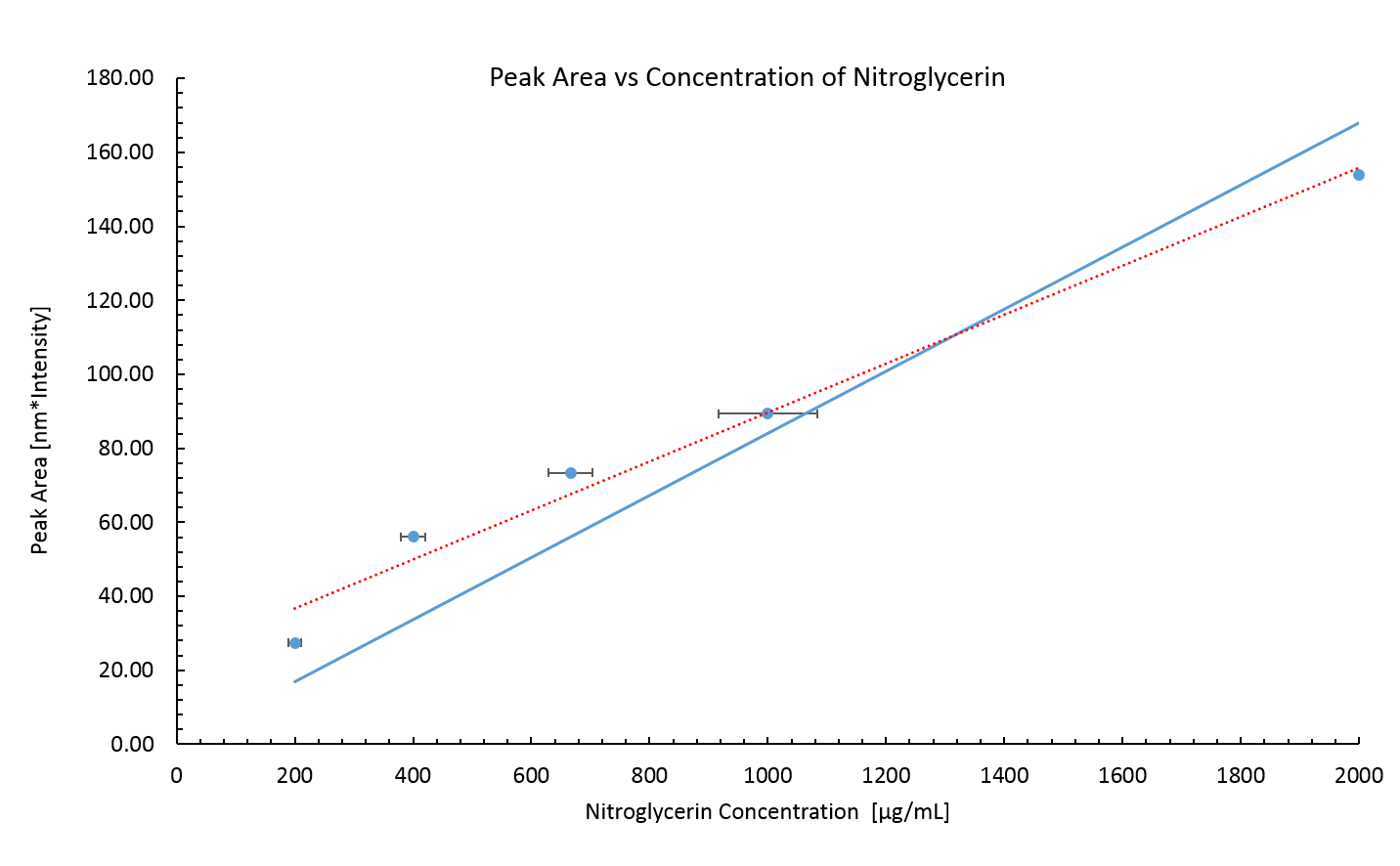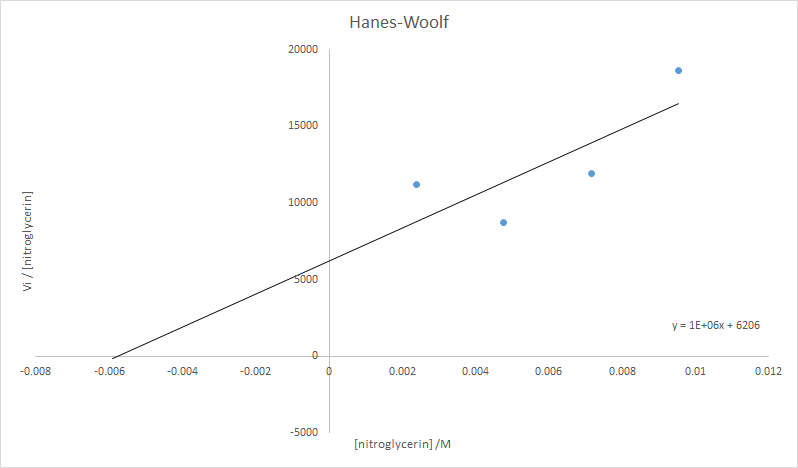Exeter | ERASE

# Validation of Enzyme Activity

## Summary

In these experiments we demonstrate that the NemA protein (encoded by BBa_K1398002) expressed as part of the construct BBa_K1398003 can catalyse the degradation of nitroglycerin. Analysis of the data collected allowed the kinetic parameters of Km and Vmax of NemA for nitroglycerin to be calculated as 6 mM and 21 umol mg$^{-1}$ min$^{-1}$ respectively. To demonstrate this we have used Raman spectroscopy to measure the concentration of nitroglycerin in aqueous solutions. For TNT please see the HPLC page.

## Abstract

NemA is a protein the Exeter iGEM team propose will allow E. coli to degrade TNT and nitroglycerin at concentrations above those normally toxic to the cell. The following experimental account describes the protocol used to confirm the substrate target of NemA and analyse the kinetic capability of this enzyme.

Raman spectroscopy is a technique used to observe vibrational, rotational and other low frequency modes in a system. It utilises the inelastic scattering of monochromatic light from these low frequency modes by detecting the shift in reflected photon energy. Inelastic scattering occurs where part of the photons energy is transferred to the vibrational state resulting in a lower energy reflected photon. This shift can be used to give information about the types of bonds in the system and how many of these are present.

Typically the inelastically reflected light from the low frequency modes in a system is very weak in comparison to the elastically scattered light from the sample. In the past this had been the major barrier to the wider use of Raman spectroscopy. With recent improvements to band-stop filters the ease of processing the signal from the sample has been greatly improved and as a result Raman spectroscopy has been put to greater use.

Raman spectroscopy was used for this experiment because of its ability to both detect the compounds of interest and give quantifiable values for their concentration. The data obtained in this experiment complements similar measurements made using high performance liquid chromatography. In addition, this experiment will provide an early indication as to whether this type of spectroscopy is suitable for use on aqueous organic samples.

## Results

### The Raman spectrum of TNT and nitroglycerin

Figure 1 shows the Raman spectrum of NG and TNT observed using undiluted samples. The NG spectrum agrees with literature spectrum having two high intensity peaks at 800cm$^{-1}$ and 840cm$^{-1}$ . The TNT spectrum corresponds to the literature spectrum  with its highest intensity peaks at 820$^{-1}$ and 1360 cm$^{-1}$.

The TNT spectrum has a low intensity in compared to the nitroglycerin. This is to be expected as the nitroglycerin sample was at a higher concentration (2mgml$^{-1}$) than the TNT sample (1mgml$^{-1}$).Figure 1: The Raman spectrum of TNT and nitroglycerin. The TNT spectrum agrees with literature values having high intensity peaks at 820cm$^{-1}$ and 1360cm$^{-1}$ . Nitroglycerin also agrees with literature values having two high intensity peaks at 800cm$^{-1}$ and 840cm$^{-1}$.

### The Standard curve

Figure 2 shows the normalised Raman spectrum for nitroglycerin at various concentrations between the wavenumbers of 850 cm$^{-1}$ and 750 cm$^{-1}$. The spectrum was normalised between 1200 cm$^{-1}$ and 600 cm$^{-1}$ by dividing by the average intensity between these points. The concentration is proportional to the peak area.Figure 2: The normalised Raman spectrum for nitroglycerin. The concentration of nitroglycerin is proportional to the peak area.

The figure 2 also shows the predicted background intensity level without the peaks. This was made by plotting a least square fitted line through measurements around the peak sites. The background intensity level increases with lower intensity peaks, this is an artifact of normalisation.

Using the predicted background intensity the peak area, and thereby the concentration, can be calculated using the trapezium rule,

\begin{equation} \text{Area} = \sum_i^{N-1} \frac{I_i+I_{i+1}}{2}(k_{i+1}-k_i). \end{equation}

Where $I_i$ is the intensity of the $i$th measurement and $k_i$ is the wavenumber of the $i$th measurement.

The peak area is plotted against the concentration of the prepared sample in figure 3. A least square fitted line (Red dashed line) does not intercept the origin of the graph. This error could be due to a systematic error in transferring the nitroglycerin into the dilutions. The stock nitroglycerin solution was quite viscous meaning that some extra nitroglycerin stuck to the outside of the pipette, this would have increased the amount of compound in each dilution.

The error in the nitroglycerin concentration attempted to account for the difficulty of mixing the correct quantities of NG and water by estimating the quantity of nitroglycerin to be $\pm$ 0.5 $\mu$l. This is probably incorrect as the actual error would be biased towards greater concentrations of nitroglycerin.

Assuming that the peak height must be zero at zero concentration another least square fitted line was plotted, this time fixing the intercept to zero. This line will be used for determining concentrations of NG in future measurements.Figure 3: Peak area of Raman spectrum for known concentrations of nitroglycerin. The nitroglycerin concentrations are probably lower than the real values due to a systematic error incurred by the viscosity of the stock nitroglycerin solution.

The error in the peak area is small, it is calculated via,

\begin{equation} \delta \text{Area} = \sigma \langle k_{i+1}-k_i \rangle \sqrt{\frac{N}{2}}, \end{equation}

where $\sigma$ is the standard deviation from the predicted background intensity of the background intensity measurements. $\langle k_{i+1}-k_i \rangle$ is the average wavenumber gap between measurements and $N$ is the number of measurements. The error in the peak area diverges from the measured area considerably for the large numbers of measurements used to calculate it.

No standard curve for TNT was made. TNT was found to be immiscible with water resulting in extremely variable peak intensities when measuring TNT/water samples. This was likely due to the TNT forming a layer on top of the water, when part this layer was deposited onto the slide the concentration would be unexpectedly high. However for most measurements only the water part of the sample would be placed onto the slide resulting in no peaks.

Due to the immiscibility of TNT and water, measurements of TNT's concentration could not be made.

### NemA Activity

The results of the control experiments (lacking a single cofactor or NemA) are shown in figure X.

These results show that all three cofactors are required for NemA to catalyse the degradation of nitroglycerin. Furthermore NemA is responsible for the decrease in nitroglycerin concentration shown in figure X.

Figure 5 shows that iGEM Exeter have produced a synthetic E. coli bacterial strain which produces a protein capable of degrading nitroglycerin!

### NemA Kinetic Analysis

Figure 6 shows NemA reaction kinetics as a function of initial velocity, Vi, against initial TNT concentration (derived from taking a tangent at the steepest section of each series in figure 5).

The data can be displayed as a Hanes plot (figure 7) to accurately determine the Km and Vmax values.Figure 6: Decrease in nitroglycerin concentration over time at different starting concentrations of nitroglycerin.

Analysis of these data allowed the kinetic parameters of Km and Vmax of NemA for nitroglycerin to be calculated:

• Km = 6 mM
• Vmax = 21 umol mg$^{-1}$ min$^{-1}$

(0.046 mg of NemA was present in each reaction mixture)

These values were used in the mathematical model we have devleoped which can be seen on the Modelling page.

## Experimental Method

### The Standard Curve

In order for the results of any concentration measurement to be analyzed a standard curve of compound concentration versus peak area must be created. To do this the compound is mixed from a known concentration with distilled water to create solutions at a variety concentrations. For nitroglycerin the concentrations were 200, 100, 66.7, 40 and 20 $\mu$gml$^{-1}$.

To measure the spectra of the compound at different concentrations 1 μl of the solution was placed onto an aluminium slide. The slide was placed into the Raman microscope. The microscope acts as the sight for the laser, a cross hair on the microscope mounted camera output shows where the laser will become incident on the droplet.

A 785nm laser was chosen to detect the carbon nitrogen bonds found in nitroglycerin and TNT . The laser was set to a power output of 33 Watts, this was a compromise between peak intensity and preventing large convection currents in the droplet from building up. Inelastic scattering was scanned between wavenumbers of 2000nm$^{-1}$ and 500nm$^{-1}$. Two scans were completed and combined to accentuate any peaks.

### Purifying NemA

A 15 mL overnight culture of Exeter iGEM Team's construct BBa_K1398003 was grown in LB over 12 hours from glycerol stock. A 10 mL sample of this overnight culture was added to 500 mL LB and incubated for 3 hours at 37 $^{\circ}$C and 200 RPM, whereupon the optical density of the culture reached 0.7. 0.5 mL IPTG was added. After four hours the culture was centrifuged at 4500 g for 20 minutes at 4 $^{\circ}$C. The resulting pellet was resuspended in Tris buffer and lysed by sonication. The whole cell lysate was centrifuged for a further 30 minutes at 4500 g to remove insoluble components. NemA was separated from the supernatent by passing the whole cell lysate through a Nickel column. NemA as encoded by part BBa_K1398003 bears a His-tag to enable Ni purification. Purified NemA was stored at 5 $^{\circ}$C and the concentration of the resulting aqueous protein was quantified by absorbance in a Qubit 2.0 Flurometer to be 0.045 mM.

### Stopped Enzyme Assay

A master mix of FMN (0.2 mM), NADH (0.2 mM), NADPH (0.2 mM) and NemA (0.009 mM) was created. Each reaction was started by addition of nitroglycerin, incubated at 20 $^{\circ}$C, to 0.131 mL of master mix. Final nitroglycerin reaction concentrations were: 9.5 mM, 7.2 mM, 4.8 mM and 2.4 mM. Samples of these reaction mixtures were taken and enzymes denatured by boiling at 1, 5, 10 and 30 minutes after addition of nitroglycerin. In conjunction with these reactions, four control experiments were also performed; involving the single removal of either FMN, NADH, NADPH and NemA. These controls demonstrated nitroglycerin degradation occurred via NemA activity.

Nitroglycerin samples were immediately sampled by Raman spectroscopy; using the method previously described.

## References

1. COLLANDER, R., AND BARLUND, H.: Acta Botan. Fennica 11, 1 (1930)
2. Trace level detection and identification of nitro-based explosives by surface-enhanced Raman spectroscopy, S. Botti,* S. Almaviva
3. Explosive Detection using the D3 Klarite SERS technology, D3 Technologies Ltd.
4. Fluorescence Background Problem in Raman Spectroscopy, Shan Yang and Ozan Akkus